Next: Physical Constants Up: Relativistic Electron Theory Previous: Positron Theory

# Exercises

1. Demonstrate that the four operators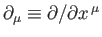transform under Lorentz transformation as the covariant components of 4-vector, whereas the four operators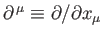transform as the contravariant components of the same vector.

2. Demonstrate that Equation (11.29) is equivalent to Equations (11.24)-(11.26).

3. Noting that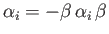, prove that the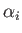and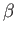matrices all have zero trace. Hence, deduce that each of these matrices has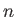eigenvalues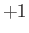, andeigenvalues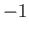, where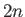is the dimension of the matrices.

4. Verify that the matrices (11.30) and (11.31) satisfy the anti-commutation relations (11.29).

5. Verify that the matrices (11.32) and (11.33) satisfy Equations (11.24)-(11.26).

6. Verify that if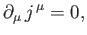where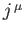is a 4-vector field, then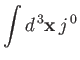is Lorentz invariant, where the integral is over all space, and it is assumed that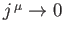as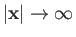.

7. Verify that Equation (11.77) is a solution of Equations (11.76).

8. A Lorentz transformation between frames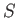and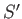takes the form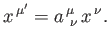If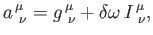where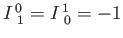, and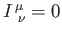otherwise, then the transformation corresponds to an infinitesimal velocity boost,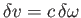, parallel to the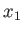-axis. Show that if a finite boost is built up from a great many such boosts then the transformation matrix becomes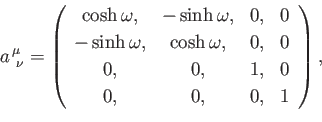where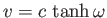is the velocity of framerelative to frame. Show that the corresponding transformation rule for spinor wavefunctions is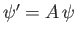, where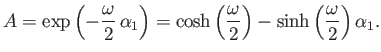9. Show that the transformation rule for spinor wavefunctions associated with a Lorentz transformation from frameto some framemoving with velocity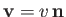with respect tois, where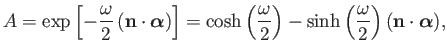and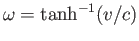.

10. Consider the spinors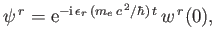for. Here,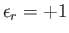for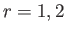, and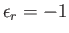for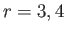. Moreover,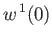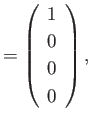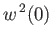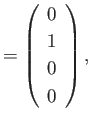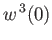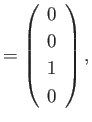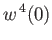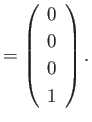Verify that the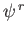are solutions of the Dirac equation in free space corresponding to electrons of energy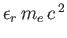, momentum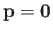, and spin angular momentum parallel to the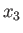-axis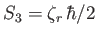, where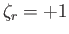for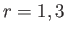, and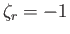for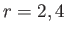.

11. Show that the four solutions of the Dirac equation corresponding to an electron of energy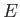and momentum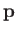moving in free space take the form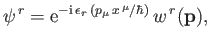where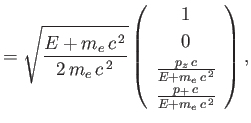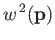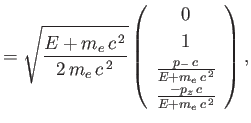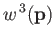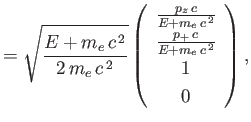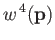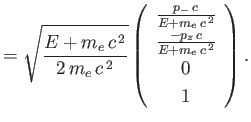Here,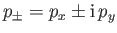. Demonstrate that these spinors become identical to theof the previous exercise in the limit that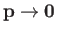.

12. Verify that the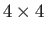matrices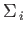, defined in Equation (11.98), satisfy the standard anti-commutation relations for Pauli matrices: that is,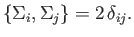Next: Physical Constants Up: Relativistic Electron Theory Previous: Positron Theory
Richard Fitzpatrick 2016-01-22